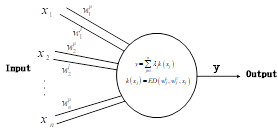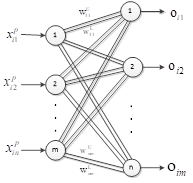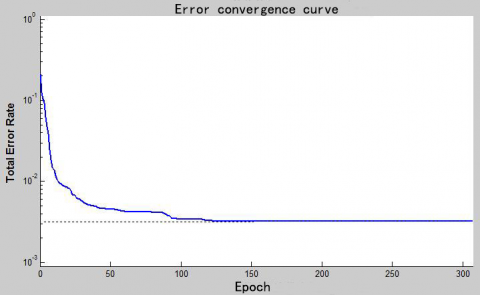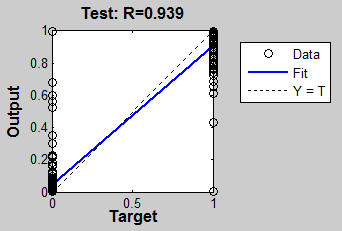# Research of Multi Sensor Information Fusion Technology Based on Extension Neural Network

Research of Multi Sensor Information Fusion Technology Based on Extension Neural Network

Tichun Wang* Yuzhu Xie Hao Yan

College of Mechanical and Electrical Engineering, Nanjing University of Aeronautics and Astronautics, Nanjing 210016, China

Corresponding Author Email:
wangtichun2010@nuaa.edu.cn
Page:
129-134
|
DOI:
https://doi.org/10.18280/mmep.030303
| |
Published:
30 September 2016
| Citation

OPEN ACCESS

Abstract:

Multi sensor information fusion technology can fuse the information from different sensors, so as to obtain more comprehensive target information, make the right judgments and improve the system's robustness. This paper proposed a kind of new reasoning method by combining the extension theory and neural network, and established fusion model based on this method. And the application of the multi-sensor information fusion technology based on this method in fire detection system is discussed. The correct identification rate of fire is in a very high level according to simulation analysis, above 93.9%. At the same time, compared with the traditional BP neural network, it shows that the extension neural network has a prominent advantage in the speed and reliability of data fusion. So it is possible to apply the extension neural network to the multi-sensor information fusion. This method has important theoretical significance and practical value for the development of the multi-sensor information fusion technology.

Keywords:

Multi-Sensor Information Fusion, Extension Theory, Extension Neural Network, Fire Detection

1. Introduction

Multi sensor information fusion technology is a kind of simulation of the human brain for dealing with complex problems comprehensively. By combining the information obtained by the multiple sensors in certain rules, we can get consistent interpretation and description of the observed objects. Sensor information fusion has two means, one is the integration of the sensor (array technology), and the other is the information fusion through the program algorithm. The general fusion algorithms include Artificial Neural Network, Bayesian Inference, Rough Set Theory and Dempster—Shafer Inference -. Wherein, the artificial neural network is notable in the information fusion for its nonlinear approximation ability. And we generally adopt the three layer perceptron model and BP algorithm. But this kind of traditional structure needs more hidden nodes, and even more hidden layer for large learning samples . In this paper, a new neural network model – extension neural network (ENN) is proposed to solve the defects of the traditional neural network and improve the information fusion capability.

Like the introduction of fuzzy mathematics into neural network, the neural network is a new kind of neural network, which introduces the extension theory to neural network. Different researchers have different emphasis on the extension neural network. And different extension neural networks have different information processing characteristics, learning rule, and purpose. It is difficult to give certain definition of an extension neural network. But the main idea is: the extension neural network is the basic element model of the extension theory, extension distance, potential value, correlation function, extension field, diamond thinking and so on. But the main idea is introduce the concept in extenics into the neural network. It makes the ENN more advantageous to handle some classes of problems than the traditional neural network or to use the extension theory alone -.

In the extension theory, the basic-element theory is more convenient for knowledge representation. The extension set and extension correlation function can describe the essence of things from the quantitative and qualitative, which is suitable for the information that is contradictory and not precise. Extension thinking method and extension reasoning is more similar to people's thinking mode. At the same time, the extension mathematics and the extension logic also have a greater expansion of the traditional mathematics and logic . But generally speaking, the extension system is lack of self-learning, parallel processing ability and adaptive ability. The neural network can study effectively from samples directly. It has a series of advantages, such as parallel computing, distributed information storage, fault tolerance and adaptive learning function. Because of these advantages, the research of neural network has attracted many researchers' interest. But the neural network is not suitable for the expression of the model of knowledge. In the training of neural network, the initial value is set to zero or random number because it could not use the existing knowledge effectively. This will increase the training time of the network or fall into local extremum. At the same time, because of the traditional neural network based on the traditional mathematics or fuzzy mathematics and the shortcomings of its own algorithm, the application is limited. Based on the above discussion, the extension theory and neural network are combined to form the extension neural network, which can absorb the advantages of the two theories, and have better performance than the single neural network system and the extension system.

2. Structure of Multi Sensor Information Fusion System

Multi sensor information fusion system has a fusion center, and complete data fusion in the center. The fusion center can have a number of levels, to achieve different levels of integration. And there can be a variety of combination modes. So the fusion system can be of different structure. It mainly can be divided into four types: centralized, decentralized, mixed type and feedback type. This paper adopts distributed information fusion method, and use ENN to realize the fusion of multi-source information. The system structure is shown as in Figure 1.

## 1.pngFigure 1. The structure of multi sensor information fusion system

The system is composed of three parts: the first part is sensor signal acquisition and data preprocessing, the second part is data processing by ENN, and the last part is identification of the signal. Different information parameters are acquired by different sensors. Then select the training sample to study and train the network according to the practical application background. After the network training, the mapping rules between the sampling parameters and output patterns are found by the weight change. Then the extension neural network can be applied to the fusion system.

3. The Algorithm of Extension Neural Network (ENN)

Extenics is an original and traverse discipline put forward by Chinese scholars in 1983. It discusses the possibility of matters’ extension and rules and methods of innovation with formalized models, which are used to solve contradictory conditions . Extenics is a cross discipline. Its basic theory is the extension theory, and its specific method is the extension method. Extension engineering is the application of extension theory and extension method in various fields. After 30 years of development, extension theory has formed a set of mature theory system, and is widely used in control and detection, artificial intelligence and computer, economic and management and other fields.

Extenics can analysis the thing in qualitative and quantitative, but with no ability of parallel computing and learning. And neural network can realize the ability of self-learning based on samples. So the extension neural network integrates the two systems and develops their advantages to form a complementary structure. On one hand, the network can be constructed by a kind of formalized language, so that the weights of the network have obvious significance. On the other hand, the learning mechanism is introduced to the accuracy and practicality of knowledge expression. The principle of ENN is shown as Figure 2.

## 2.pngFigure 2. The principle of ENN

3.1 Structure design and algorithm description of extension neural network

The network structure of extension neural network is composed of input layer and output layer. The input layer node receives the input mode, and the number of input nodes is determined by the characteristic number of the input feature vector. The output layer is the result of the classification. The number of output layer nodes is determined by the class number. The input layer and the output layer is connected with a double weight connection. One weight value represents the minimum value of the classical domain, and the other is the upper bound of the classical domain -.

3.1.1 The extension neuron

The basic processing unit of artificial neural networks is called neuron. And the neuron model of ENN is called extension neuron. It is a simulation of biological neurons and has a structure different from other neuron constructed by the quantitative analysis tool of correlation function in extenics, shown as Figure 3.

## 3.pngFigure 3. Extension neron model

The neuron unit composed of multiple inputs ${{x}_{i}},\ i=1,2,...,n$ and one output$y$. To establish triple according to matter-element theory for each input signal, that is to establish the input signal matter-element, shown as follow:

${{N}_{i}}=\left[ s,{{x}_{i}},{{v}_{i}} \right],\ i=1,2,...,n$     (1)

Wherein, ${{N}_{i}}$ represent the ith element of input signal. $s$ represent input signal. ${{v}_{i}}$ represent the value of the ith input signal ${{x}_{i}}$. The intermediate state is represented by classic domain ${{W}_{i}}=\left[ w_{i}^{l},w_{i}^{u} \right],i=1,2,...,n$ of input signal matter-element and modification value. And the output formula is:

$y=\sum\limits_{j=1}^{n}{{{\lambda }_{j}}k\left( {{x}_{j}} \right)}$     (2)

Wherein: $k({{x}_{j}})=ED\left( w_{j}^{l},w_{j}^{u},{{x}_{j}} \right)$

$k({{x}_{j}})=\left\{ \begin{matrix} \frac{-\rho \left( {{x}_{i}},{{w}_{i}} \right)}{\left| {}^{\left( w_{kj}^{u}-w_{kj}^{l} \right)}/{}_{2} \right|} & {{x}_{i}}\in {{W}_{i}} \\ \frac{\rho \left( {{x}_{i}},{{w}_{i}} \right)}{\rho \left( {{x}_{i}},\left( v_{i}^{L}-\eta v_{i}^{L},v_{i}^{U}+\eta v_{i}^{U} \right) \right)-\rho \left( {{x}_{i}},{{W}_{kj}} \right)} & {{x}_{_{i}}}\notin {{W}_{i}} \\\end{matrix} \right.$     (3)

$\ \rho \left( {{x}_{i}},\left( w_{i}^{l},w_{i}^{u} \right) \right)=\left| {{x}_{i}}-{}^{\left( w_{i}^{l}+w_{i}^{u} \right)}/{}_{2} \right|-{}^{\left( w_{i}^{u}-w_{i}^{l} \right)}/{}_{2}$     (4)

\begin{align} & \rho \left( {{x}_{i}},\left( v_{i}^{L}-\eta v_{i}^{L},v_{i}^{U}+\eta v_{i}^{U} \right) \right) \\ & =\left| {{x}_{i}}-\frac{v_{i}^{L}-\eta v_{i}^{L}+v_{i}^{U}+\eta v_{i}^{U}}{2} \right|-\frac{v_{i}^{U}+\eta v_{i}^{U}-v_{i}^{L}+\eta v_{i}^{L}}{2} \\\end{align}     (5)

And ${{\lambda }_{j}},j=1,2,...,n$ is the corresponding weight coefficient of each characteristic.

3.1.2 The network structure of extension neuron

The structure of ENN is shown as Figure 4. It is composed of two layers of neural network, including the input layer, output layer and the link weight that connect the input neurons and output neurons. Each neuron of input layer corresponding to the different characteristics of multidimensional matter-element. And the neuron of output layer refers to the probability of fire in different stage. There are two link weights between each input layer neurons and output layer neurons. A weight is the upper bound of the value of one characteristic corresponding with one fire stage, and another weight is the lower bound of that. The upper bound and lower bound between the jth input neuron and the kth output neuron are represented as $W_{kj}^{U}$ and$W_{kj}^{L}$. And the function of output layer neuron is dependent function.

## 4.pngFigure 4. The structure diagram of ENN

3.2 The ENN algorithm based on supervision algorithm

The training pattern set is $X=\left\{ {{X}_{1}},{{X}_{2}},...,{{X}_{{{N}_{P}}}} \right\}$, ${{N}_{p}}$ is amount of training pattern. The ith pattern denoted by $X_{i}^{P}=\left\{ X_{i1}^{P},X_{i2}^{P},...,X_{in}^{P} \right\}$, $n$ is amount of characteristic. The learning error is ${{E}_{T}}=\frac{{{N}_{m}}}{{{N}_{P}}}$, ${{N}_{m}}$ is the total training error count. The specific learning algorithm steps are as follows:

Step 1: To establish the matter-element model of the weight of ENN input node and output node based on mater-element theory. As shown below:

${{R}_{k}}=\left[ \begin{matrix} {{N}_{k}} & {{c}_{1}} & \left( w_{k1}^{L},w_{k1}^{U} \right) \\ {} & {{c}_{2}} & \left( w_{k2}^{L},w_{k2}^{U} \right) \\ {} & ... & ... \\ {} & {{c}_{n}} & \left( w_{kn}^{L},w_{kn}^{U} \right) \\\end{matrix} \right],k=1,2,...,{{n}_{c}}$     (6)

Wherein, $w_{ki}^{L}$ and $w_{ki}^{U}$ represent the upper bound and lower bound of the value of the ith characteristic ${{c}_{i}}$ about the kth cluster, and $i=1,2,...,n$. The classical domain can be achieved from the given training data set.

$w_{kj}^{L}=Min\sum\nolimits_{i\in {{N}_{P}}}{\left\{ x_{ij}^{k} \right\}}$     (7)

$w_{kj}^{U}=Max\sum\nolimits_{i\in {{N}_{P}}}{\left\{ x_{ij}^{k} \right\}}$     (8)

Step 2: To calculate initial center of each clustering.

${{Z}_{k}}=\left\{ {{z}_{k1}},{{z}_{k2}},...,{{z}_{kn}} \right\}$     (9)

${{z}_{kj}}=\frac{w_{kj}^{L}+w_{kj}^{U}}{U}$     (10)

Wherein, $k=1,2,...,{{n}_{c}}$, $j=1,2,...,n$.

Step 3: Read in the pth sample of the ith training model.

$X_{i}^{p}=\left\{ x_{i1}^{p},x_{i2}^{p},...,x_{in}^{p} \right\},p\in {{n}_{c}}$     (11)

Step 4: To calculate the correlation degree of training sample $X_{i}^{p}$ and the kth cluste based on extension distance function.

$E{{D}_{ik}}=\sum\limits_{j=1}^{n}{\left[ \frac{\left| x_{ij}^{p}-{{z}_{kj}} \right|-{}^{\left( w_{kj}^{U}-w_{kj}^{L} \right)}/{}_{2}}{\left| {}^{\left( w_{kj}^{U}-w_{kj}^{L} \right)}/{}_{2} \right|}+1 \right]}$     (12)

Wherein, $k=1,2,...,{{n}_{c}}$.

Step 5: Find${{k}^{*}}$, meeting$E{{D}_{i{{k}^{*}}}}=Min\left\{ E{{D}_{ik}} \right\}$, and enter the Step7, or in Step6.

Step 6: Update the weights and clustering center.

Step 6.1: Update the clustering center of the pth sample and ${{k}^{*}}$.

$z_{pj}^{new}=z_{pj}^{old}+\eta \left( x_{ij}^{p}-z_{pj}^{old} \right)$     (13)

$z_{{{k}^{*}}j}^{new}=z_{{{k}^{*}}j}^{old}-\eta \left( x_{ij}^{p}-z_{{{k}^{*}}j}^{old} \right)$     (14)

Step 6.2: Update the weight of the pth sample and ${{k}^{*}}$.

$\left\{ \begin{matrix} w_{pj}^{L\left( new \right)}=w_{pj}^{L\left( old \right)}+\eta \left( x_{ij}^{p}-z_{pj}^{old} \right) \\ w_{pj}^{U\left( new \right)}=w_{pj}^{U\left( old \right)}+\eta \left( x_{ij}^{p}-z_{pj}^{old} \right) \\\end{matrix} \right.$     (15)

$\left\{ \begin{matrix} w_{{{k}^{*}}j}^{L\left( new \right)}=w_{{{k}^{*}}j}^{L\left( old \right)}+\eta \left( x_{ij}^{p}-z_{{{k}^{*}}j}^{old} \right) \\ w_{{{k}^{*}}j}^{U\left( new \right)}=w_{{{k}^{*}}j}^{U\left( old \right)}+\eta \left( x_{ij}^{p}-z_{{{k}^{*}}j}^{old} \right) \\\end{matrix} \right.$     (16)

Wherein, $\eta$ is the learning rate.

Step 7: Repeat step3-step6 until all model training complete, then the learning to complete.

Step 8: If the clustering process is convergent and total error reach to the given value, then training to complete, or back to step 3.

4. Application Example

In order to verify the validity and reliability of the application of extension neural network in multi sensor information fusion, this paper applied the multi sensor information fusion system based on extension neural network to the fire detection, and use the Matlab software to train and simulate it. The experimental parameters of the national standard test fire and the initial fire are used as training samples of ENN. The input of the ENN is the environmental temperature, smoke concentration and CO gas concentration, and the expected output is three kinds of fire hazard levels: L, M and H.

4.1 Data processing

The temperature range of the input information is 30 ~200, and the range of CO is 0ppm~100ppm, and the smoke concentration range is 0ppm~200ppm. The physical quantities of the network input are different, and the quantity is far from the same. So the sample data must be normalized before the network training, so as to prevent the small numerical information flooded by the large numerical value. That is to normalized the input information to [0, 1]. Take 100 sets of experimental data as training sample of the network after normalized. Some experimental data are shown in table 1.

When the fire probability (P = smoldering fire probability + fire probability) is greater than 0. 7, fire danger level is H, can be judged to be a fire; when the fire probability is less than 0. 3, fire danger level is L, can be judged to be no fire. It is more difficult to determine the fire information when the fire probability in the vicinity of 0. 5. Smoke is very important information in the early fire. Therefore, this paper introduces the smoke duration function. as the basis for the occurrence of fire. The function is shown in follows:

$d\left( n \right)=\left[ d\left( n-1 \right) \right]\mu \left[ A\left( x \right)-{{T}_{d}} \right]$     (17)

Wherein, $\mu \left[ {} \right]$ is unit step function, ${{T}_{d}}$ is judging threshold, in this paper, we take 30S. When the smoke duration is over${{T}_{d}}$, $d\left( n \right)$ is added, otherwise$d\left( n \right)=0$. $n$ is discrete time variable. Decision rules are as follows:

When $P\le 0.3$, no fire;

When $P\ge 0.7$, have a fire;

When $0.3<P<0.7$ and $d\left( n \right)>0$, have a fire;

When $0.3<P<0.7$ and $d\left( n \right)=0$, no fire, delay ${{T}_{d}}$and then judge.

Table 1. Training sample of the network

 NO. Input data Expected output probability Fire danger level Temperature Smoke concen-stration CO concen-tration No fire Smoldering fire Open fire 1 0. 2 0. 42 0. 48 0. 1 0. 6 0. 3 H 2 0. 45 0. 18 0. 75 0. 2 0. 3 0. 5 H 3 0. 95 0. 2 0. 75 0 0. 2 0. 8 H 4 0. 5 0. 55 0. 7 0. 15 0. 3 0. 55 H 5 0. 14 0. 1 0. 52 0. 65 0. 25 0. 1 M 6 0. 1 0. 52 0. 14 0. 65 0. 25 0. 1 M 7 0. 42 0. 2 0. 1 0. 68 0. 2 0. 12 M 8 0. 15 0. 08 0. 23 0. 76 0. 2 0. 04 L 9 0. 03 0. 14 0. 4 0. 85 0. 1 0. 05 L 10 0. 1 0. 14 0. 2 0. 89 0. 05 0. 06 L

4.2 Network training

Firstly, the matter element model of three different fire hazard levels are established, which are L (${{N}_{1}}$), M (${{N}_{2}}$), H (${{N}_{3}}$) . Its characteristics are three input signals: the temperature (${{c}_{1}}$), smoke concentration (${{c}_{2}}$) and CO concentration (${{c}_{3}}$). Then determine the range of the material element's segment according to the sample data.

Table 2. Matter element model of fire hazard level

 Fire hazard level Matter element model L ${{R}_{1}}=\left( {{N}_{1}},C,{{v}_{1}} \right)=\left[ \begin{matrix} {{N}_{1}} & {{c}_{1}} & \left\langle 0\tilde{\ }0.625 \right\rangle \\ {} & {{c}_{2}} & \left\langle 0\tilde{\ }0.52 \right\rangle \\ {} & {{c}_{3}} & \left\langle 0\tilde{\ }0.63 \right\rangle \\\end{matrix} \right]$ M ${{R}_{2}}=\left( {{N}_{2}},C,{{v}_{2}} \right)=\left[ \begin{matrix} {{N}_{2}} & {{c}_{1}} & \left\langle 0.1\tilde{\ }0.67 \right\rangle \\ {} & {{c}_{2}} & \left\langle 0.1\tilde{\ }0.69 \right\rangle \\ {} & {{c}_{3}} & \left\langle 0.14\tilde{\ }0.66 \right\rangle \\\end{matrix} \right]$ H ${{R}_{3}}=\left( {{N}_{3}},C,{{v}_{3}} \right)=\left[ \begin{matrix} {{N}_{3}} & {{c}_{1}} & \left\langle 0.1\tilde{\ }1 \right\rangle \\ {} & {{c}_{2}} & \left\langle 0.13\tilde{\ }1 \right\rangle \\ {} & {{c}_{3}} & \left\langle 0.1\tilde{\ }1 \right\rangle \\\end{matrix} \right]$

To initial network weights according to the matter element model. Then the network training is carried out according to the above algorithm. The error convergence curve is shown in Figure 5. Here the learning rate is 0. 1.

## 5.pngFigure 5. Error convergence curve

The system automatically assigns certain data as test data. Test accuracy curve is shown in Figure 6.

## 6.pngFigure 6. Test accuracy curve based on ENN network

4.3 Comparative analysis

Table 3. Experimental comparison results

 Network model ENN model BP neural network model Network structure 3-3(2 layers) 3-8-3(3 layers) Learning rate 0. 1 Variable learning rate Number of connection weights 18 72 Training epoch 125 175 Training error 0. 0051 0. 0069 Test accuracy(%) 93. 9 83

In order to verify the superiority of the proposed method in this paper, the method is compared with the method in literature . The method in literature  adopts BP neural network. Through the comparison, ENN model and BP neural network model can be effective for fire warning, but the ENN model improved greatly in the network structure, learning performance and learning time, as shown in table 3. On the network structure, the BP neural network model has three layers: the input layer, the hidden layer and the output layer. The number of neurons in each layer are 3, 8 and 3, and the number of connection weights is 72; the ENN model only has the input layer and the output layer the connection weight number is 18. In the learning performance, the learning error of the ENN model converges to 0. 0051 after 125 steps of training, and the learning error of BP neural network model converges to 0. 0069 after 175 steps. Thus, the fire warning method based on ENN not only meets the practical needs, but also improves the performance.

5. Conclusions

In this paper, we describe the network structure, algorithm process and experimental study of the extension neural network. The validity and superiority of the method are verified by experiments. Through experiment comparison, it can be seen that the method has the advantages of simple design, simple structure, fast convergence and smaller training error, which can greatly improve the performance of the data fusion system, and provide a new method and idea for multi sensor information fusion.

Acknowledgment

This research was supported by the National Natural Science Foundation Youth Fund of China (No. 51005114); The Fundamental Research Funds for the Central Universities, China (No. NS2014050); The Research Fund for the Doctoral Program of Higher Education China(No. 20112302130003); Jiangsu Planned Projects for Postdoctoral Research Funds (No. 1301162C).

References

 He You, Wang Guo Hong and Lu Dajin, Multi Sensor Information Fusion and Its Application, Beijng: Electronics Industry Press, 2000.

 Wang Yaonan and Li Shutao, “Multi-sensor Information Fusion and Its Application: A survey,” Control and Decision, vol. 16, no. 5, pp. 518-522, 2001.

 Pan Quan, Yu Xin, Cheng Yong mei. Essential Methods and Progress of Information Fusion Theory. Acta automatica sinica, vol. 29, no. 4, pp. 599-615, 2003.

 Zhao Rui, He Jianjun, “Technology of multisensory information fusion,” Computer Measurement & Control, no. 9, pp. 124–127, 2007.

 Yang Fan, Chen Maolin, Wu Xun, et al., “Research of multi-sensor information fusion based on BP neural networks,” Computer Measurement & Control, vol. 23, no. 5, pp. 1823-1826, 2015.

 Zhou Yu, Qian Xu, Zhang Jun Cai, “Overview of extension neural network,” Application Research Of Computers, vol. 27, no. 1, pp. 1-5, 2010.

 Feng Jing, “Application of extension theory in the research of RBF neural network,” Beijing: Beijing University of Chemical Technology, 2011.

 Yang Chunyan, Cai Wen, Extension Engineering, Beijing: Science Press, 2007.

 Cai Wen, “Extension Management Engineering and Application,” International Journal of Operations and Quantitative Management, no. 1, pp. 59-72, 1999.

 Sun Baiqing, Xing Aiguo, Zhang Jibin, et al., “Design and implementation neural network model,” Journal of Harbin Institute of Technology, no. 7, pp. 1156-1159, 2006.

 M. H. Wang and C. P. Hong, “Extension neural network,” Proc. Int. Joint Conf. Neural Networks, pp. 399-403, 2003.

 M. H. Wang, “Extension neural network and its application,” Neural Networks, vol. 16, pp. 779-784, 2003.

 Zhou Xiaolin, “Research on fire alarm system based on multi-sensors data fusion of neural network,” Changchun University of Technology, Changchun, China, 2012.